# Chapter 10: Stage and Contineous Gas-Liquid Seaparation Procesess¶

## Example 10.2-1 Page Number 587¶

In :
#Dissolved Oxygen Concentration in Water

#Variable Declaration
H = 4.38e4                   #Henry's law constant atm/mol fraction
yO2 = 0.21                   #mol fraction

#Calculations
#y = Hx
xO2 = yO2/H

#Results
print 'Mol fraction of oxygen in water, xA: %5.3e'%xO2

Mol fraction of oxygen in water, xA: 4.795e-06


## Example 10.3-1 Page Number 589¶

In :
#Equilibrium Stage Contact for CO2-Air-Water
from scipy.optimize import root

#Variable Declaration
P = 1.            #Absolute pressure of gas mixture (atm)
yA2 = 0.2         #Mole fraction of CO2
Vm = 100.         #Feed inlet
Lm = 300.         #Flowarte of liquid entering (kg mol water/hr)
xC0 = 1.
xB0 = 0.
xA0 = 0
H = 0.142e4       #Henry's law constant from appendix A.3 in atm/mol frac

#Calculations
Lmd = Lm
Vmd = (1-yA2)*Vm
Hd = H/P
#Lmd*xA0/(1-xA0) + Vmd*yA2/(1-yA2) = Lmd*xA1/(1-xA1) + Vmd*yA1/(1-yA1)
#yA1 = Hd* xA1
RHS = (Vmd*yA2)/(1-yA2)
f = lambda xA1: Lmd*xA1/(1-xA1) + Vmd*Hd*xA1/(1-Hd*xA1)-Vmd*yA2/(1-yA2)
sol = root(f, 0.0001)
xA1 = sol.x
#Results
yA1 = Hd*xA1
L1 = Lmd/(1-xA1)
V1 = Vmd/(1-yA1)

print 'Outlet liquid and vapor phase compositions are xA1: %5.2e and yA1: %4.2f'%(xA1,yA1)
print 'Liquid and vapor rates from colums are L1:%4.1f kgmol/h V1: %4.1f kgmol/h respectively'%(L1,V1)

Outlet liquid and vapor phase compositions are  xA1: 1.41e-04 and yA1: 0.20
Liquid and vapor rates from colums are L1:300.0 V1: 100.0 respectively


## Example 10.3-2 Page Number 591¶

In :
#Absorption of Acetone in a Countercurrent Stage Tower
import numpy as np
import matplotlib.pyplot as plt

#Variable Declaration
VN1 = 30.       #Inlet Gas Flow Rate, kg mol/hr
L0 = 90.        #Inlet Liquid Flow Rate, kg mol/hr
xA0 = 0.0       #Mole fraction of Acetone in entering Water
yAN1 = 0.01     #Mole fraction of Acetone in entering Air
AAAds = 90.     #Percent of acetone absorbed
H = 2.53

#Calculations
# moles of acetone entering

AVi = yAN1*VN1
Ai = (1-yAN1)*VN1
AVo = (1-AAAds/100)*AVi
ALo = AAAds*AVi/100
V1 = AVo + Ai
yA1 = AVo/Ai
LN = L0 + ALo
xAN = ALo/LN

y = np.arange(0,0.012,0.0005)
x = y/H

plt.plot(x,y)
plt.text(.0035, .007, r'Equilibrium Curve')
plt.text(.001, .009, r'Operating Line')
plt.plot(xAN,yAN1,'ro')
plt.annotate('$(x_{AN},y_{AN+1})$', xy=(xAN,yAN1), xytext=(xAN,yAN1))
plt.plot(xA0,yA1,'ro')
plt.annotate('$(x_{A0},y_{A1})$', xy=(xA0,yA1), xytext=(xA0,yA1+0.001))

m = (yAN1-yA1)/(xAN-xA0)

def ypos(xop):
return m*xop + yA1

def xpos(yop):
return yop/H

yy = m*x + yA1
plt.plot(x,yy,'r-')
plt.xlabel('Mole fraction of acetone in water, $x_A$')
plt.ylabel('Mole fraction of acetone in air, $y_A$')
plt.plot([xAN,xAN],[0.0,yAN1],'b--')
plt.plot([0.0,xAN],[yAN1,yAN1],'b--')
x1 = xA0
y1 = yA1
n = 0
while x1 <= xAN:
x2 = xpos(y1)
y2 = y1
if x2 > xAN:
plot([x1,x2], [y1,y2], 'k-', lw=1)  #Draw Horizontal line to equilibrium curve
dxt = x2-x1
dx = xAN-x1
dxbydxt = dx/dxt
n = n + dxbydxt
break
plt.text(x2, y2-0.0007, str(n+1))
plot([x1,x2], [y1,y2], 'k-', lw=1)      #Draw Horizontal line to equilibrium curve
x1 = x2
y1 = y2
y2 = ypos(x1)
plot([x1, x2], [y1, y2], 'k-', lw=1)    #Draw a vertical line to operating line
n = n+1
x1 = x2
y1 = y2

print "Acetone laden air rate from the absorber:",V1, "kgmol air/hr"
print "Acetone concentration in leaving stream", round(yA1,6)
print "Acetone + Water rate from the absorber:", LN, "kmol/hr"
print "Acetone concentrqtion in liquid leaving absorber:",round(xAN,5)
print "Amount of acetone etering with air", round(AVi,4),"kmol acetone/hr"
print "Amount of air entering ", round(Ai,4), " kgmol air/hr"
print "Amount of acetone leaving with treated air", round(AVo,4), "kgmol/hr"
print "Amount of acetone leaving with Water", round(ALo,4), "kgmol/hr"
print "Number of Stages:", round(n,1)

Acetone laden air rate from the absorber: 29.73 kgmol air/hr
Acetone concentration in leaving stream 0.00101
Acetone + Water rate from the absorber: 90.27 kmol/hr
Acetone concentrqtion in liquid leaving absorber: 0.00299
Amount of acetone etering with air 0.3 kmol acetone/hr
Amount of air entering  29.7  kgmol air/hr
Amount of acetone leaving with treated air 0.03 kgmol/hr
Amount of acetone leaving with Water 0.27 kgmol/hr
Number of Stages:  5.1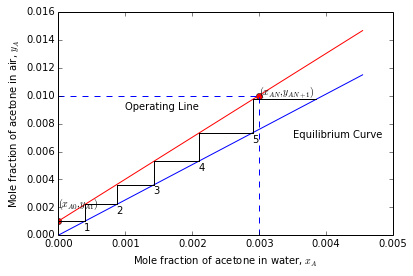## Example 10.3-3 Page Number 593¶

In :
#Number of Stages by Analytical Equation

import numpy as np

#Variable Declaration
VN1 = 30.       #Inlet Gas Flow Rate, kg mol/hr
L0 = 90.        #Inlet Liquid Flow Rate, kg mol/hr
xA0 = 0.0       #Mole fraction of Acetone in entering Water
yAN1 = 0.01     #Mole fraction of Acetone in entering Air
AAAds = 90.     #Percent of acetone absorbed
m = 2.53

#Calculations
# moles of acetone entering
AVi = yAN1*VN1
Ai = (1-yAN1)*VN1
AVo = (1-AAAds/100)*AVi
ALo = AAAds*AVi/100
V1 = AVo + Ai
yA1 = AVo/Ai
LN = L0 + ALo
xAN = ALo/LN

A1 = L0/(m*V1)
AN = LN/(m*VN1)
Agm = sqrt(A1*AN)

N = log((yAN1-m*xA0)/(yA1-m*xA0)*(1-1/Agm)+1/Agm)/log(Agm)

#Results
print "Geometric mean Absorption Factor:", round(Agm,3)
print "Number of stages required for separation:", round(N,2)

Geometric mean Absorption Factor: 1.193
Number of stages required for separation: 5.05


## Example 10.4-1 Page Number 597¶

In :
#Number of Stages by Analytical Equation

import numpy as np
from scipy.interpolate import interp1d
from scipy.optimize import root

#Variable Declaration
yAG = 0.380       #Inlet Gas Flow Rate, kg mol/hr
xAL = 0.100       #Inlet Liquid Flow Rate, kg mol/hr
ky = 1.465e-3     #Gas phase mass transfer coefficient, kmol/(m2.s)
kx = 1.967e-3     #Gas phase mass transfer coefficient, kmol/(m2.s)
xA = np.array([0.00,0.05,0.10,0.15,0.20,0.25,0.30,0.35])
yA = np.array([0.00,0.022,0.052,0.087,0.131,0.187,0.265,0.385])

#Calculations
f = interp1d(xA, yA, kind='cubic')
y = f(xA)
plot(xA,yA,'ro-')
#(1-xA)iM = 1., (1-yA)iM = 1.
#Slope of operating line -(kxd/(1-xA)iM )/(kyd/(1-yA)iM)
m = -kx/ky
c = yAG - m*xAL
plot(xAL, yAG,'bo')
xlabel('$mol\ fraction\ in\ liquid\ phase,\ x_A$')
ylabel('$mol\ fraction\ in\ gas\ phase,\ y_A$')
ff = lambda x: f(x) - (m*x+c)
def lm(a,b):
return ((1-a)-(1-b))/log((1-a)/(1-b))

def flux(k,a,b):
return k*(a-b)/lm(a,b)

n = 4
er = 1.0
i=0
while er>= 0.065:
i+=1
sol = root(ff, 0.002)
xAi = sol.x
yAi = f(xAi)
plot(xAi, yAi,'o-',label=str(i))
plot([xAL,xAi], [yAG, yAi], lw=0.3)
ylm = lm(yAi,yAG)
xlm = lm(xAi,xAL)
mc = -kx/xlm/(ky/ylm)
NAy = flux(ky,yAG,yAi)
NAx = flux(kx,xAi,xAL)
#er = abs((NAx-NAy)/NAx)
er = abs((m-mc)/m)
m = (n*m + mc)/(n+1)
legend(loc='lower right')
#Results
print "Liquid phase Interphase concentration:", round(xAi,3)
print "Gas  phase  Interphase  concentration:", round(yAi,3)
print 'Flux  in  Gas  phase: %4.2e'%(NAy)
print 'Flux in Liquid phase: %4.2e'%(NAx)

Liquid phase Interphase concentration: 0.257
Gas  phase  Interphase  concentration: 0.196
Flux  in  Gas  phase: 3.80e-04
Flux in Liquid phase: 3.78e-04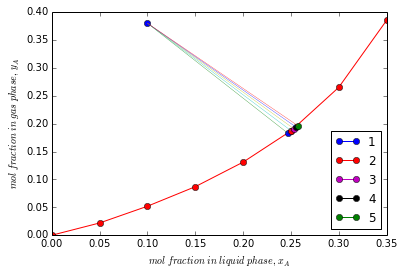## Example 10.4-2 Page Number 601¶

In :
#Overall Mass Transfer Coefficients from Film Coefficients
import numpy as np
from scipy.interpolate import interp1d
from scipy.optimize import root

#Variable Declarion
yAG = 0.380       #Inlet Gas Flow Rate, kg mol/hr
xAL = 0.100       #Inlet Liquid Flow Rate, kg mol/hr
ky = 1.465e-3     #Gas phase mass transfer coefficient, kmol/(m2.s)
kx = 1.967e-3     #Gas phase mass transfer coefficient, kmol/(m2.s)
xA = np.array([0.00,0.05,0.10,0.15,0.20,0.25,0.30,0.35])
yA = np.array([0.00,0.022,0.052,0.087,0.131,0.187,0.265,0.385])
xAi = 0.2572
yAi = 0.1964

f = interp1d(xA, yA, bounds_error=False, fill_value=-10)

def flux(k,a,b):
return k*(a-b)/lm(a,b)

def lm(a,b):
return ((1-a)-(1-b))/log((1-a)/(1-b))

#f2 = lambda x: f(x)- yAG #sol = root(f2, 0.3, method='hybr') xAs = sol.x print xAs
yAs = f(xAL)
md = (yAi-yAs)/(xAi-xAL)
yLMs = lm(yAs,yAG)
yLM = lm(yAi,yAG)
xLM = lm(xAi,xAL)
InvKydby_yLMsGas = 1/(ky/yLM)
InvKydby_yLMsLiquid =  md/(kx/xLM)
InvKydby_yLMs = InvKydby_yLMsGas + InvKydby_yLMsLiquid
Kyd = (1/InvKydby_yLMs)*yLMs
Rgasper = InvKydby_yLMsGas*100/InvKydby_yLMs
Rliquidper = InvKydby_yLMsLiquid*100/InvKydby_yLMs
NA = Kyd*(yAG-yAs)/yLMs
#Results
print "Individual Film resistances for Gas and Liquid film are:", round(InvKydby_yLMsGas,2),round(InvKydby_yLMsLiquid,2), "respectively"
print 'Overall Mass Transfer Coefficient: %5.3e'%(Kyd), "kgmol/(m2.s)"
print "Percentage Resistance in Gas and Liquid film is:", round(Rgasper,1),"&", round(Rliquidper,1),"respectively"
print 'The Flux of A is: %5.3e'%(NA), "kgmol/(m2.s)"

Individual Film resistances for Gas and Liquid film are: 483.16 382.41 respectively
Overall Mass Transfer Coefficient: 8.924e-04 kgmol/(m2.s)
Percentage Resistance in Gas and Liquid film is: 55.8 & 44.2 respectively
The Flux of A is: 3.789e-04 kgmol/(m2.s)


## Example 10.5-1 Page Number 608¶

In :
#Design of Water-Cooling Tower Using Film Coefficients
import numpy as np
import matplotlib.pyplot as plt
from scipy.interpolate import interp1d
from scipy.optimize import root
import scipy.integrate as integrate

#Variable Declarion
P = 101325      #Atmospheric pressure
G = 1.356       #Inlet Gas Flow Rate, kg mol/hr
L = 1.356       #Inlet Liquid Flow Rate, kg mol/hr
TL1 = 29.4      #Initial temperature of water in °C
TL2 = 43.3      #Final temperature of water in °C
TG1 = 29.4      #Inlet air temperature of air in °C
Tw  = 23.9      #Wet bulb temperature of air in °C
kGa = 1.207e-7  #Mass Transfer Coefficient, kmol/(m2.s.Pa)
K = 4.187e4     #J/(kg.K)
cL = 4187.       #J/(kg.K)
T0 = 0.0
H1 = 0.0165     #Humidity of entering air, kgWater/kgDryAir
Mb = 29.

#Calculation
t = np.array([15.6,26.7,29.6,32.2,35.0,37.8,40.6,43.3,46.1])
H  = np.array([43.68e3,84.0e3,97.2e3,112.1e3,128.9e3,148.2e3,172.1e3,197.2e3,224.5e3])

f = interp1d(t,H,kind='cubic',bounds_error=False)
T = np.arange(28.,46.,1)
Hy = f(T)

Hy1 = (1.005+1.88*H1)*1e3*(TG1-0.0)+2.501e6*H1
Hy2 = L*cL*(TL2-TL1)/G + Hy1
plt.figure(1)
plt.plot(t,H,'k-',T,Hy,'k-')
plt.plot([TL1,TL2],[Hy1,Hy2],'ro-')
plt.xlabel('$Liquid Temperature, C$')
plt.ylabel('$Enthalpy Hy, J/kg$')

m1=(Hy2-Hy1)/(TL2-TL1)
c1=Hy1-m1*TL1
m2 = K
TT = linspace(TL1,TL2,11)
plt.xlim(25,45)
plt.ylim(40000,200000)
Hyi = ()
Hy = ()
cnt = 0
for i in TT:
y1 = m1*i+c1
c2 = y1-m2*i
ff = lambda xx: f(xx)-y1+K*(xx-i)
sol = root(ff,40)
x2 = sol.x
y2 = f(x2)
Hy = np.append(Hy,y1)
Hyi = np.append(Hyi,y2)
cnt = cnt + 1
plt.plot([i,x2],[y1,y2],'o-')
InvHyimHy = 1/(Hyi-Hy)

z = (G/(Mb*kGa*P))*integrate.simps(InvHyimHy,Hy)
plt.figure(2)
plt.plot(Hy,InvHyimHy)
plt.fill_between(Hy,InvHyimHy,0,color='0.6')
plt.text(90000, 0.00002, 'Area = '+str(round(z,2))+' m')
plt.xlabel('$Hy$')
plt.ylabel('$1/(Hyi-Hy)$')
plt.show()
#Results
print "Height of packed cooling tower:", round(z,2),"m"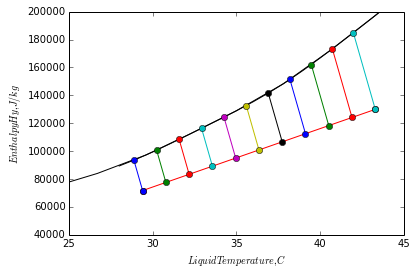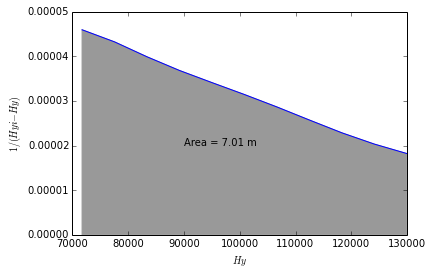Height of packed cooling tower: 7.01 m


## Example 10.6-1 Page Number 614¶

In :
#Absorption of SO2 in Plate Tray
from scipy.optimize import root
from scipy.interpolate import interp1d
import matplotlib.pyplot as plt
import numpy as np

#Variable Declaration
ysiP = 0.20   #Mole fraction of SO2 entering
ysoP = 0.02   #Mole fraction of SO2 leaving
P = 101325.   #Total pressure at which SO2 is leaving in Pa
Fair = 150    #Flowrate of air in kg/hrm2
Fwat = 6000   #Flowrate of water in kg/hrm2
effP = 0.25   #Fractional efficiency
MWair = 29    #Molecular weight of air
MWW = 18      #Molecular weight of water
x = np.array([0.0000,0.0005,0.001,0.0015,0.002,0.0025,0.003,0.0035,0.004,0.0045,0.005,0.0055,0.006])
y = np.array([0.,0.,0.,0.,0.,0.,0.,0.,0.,0.,0.,0.,0.])
xe = np.array([0.0,0.0000562,0.0001403,0.000280,0.000422,0.000564,0.000842,0.001403,0.001965,0.00279,0.00420,0.00698])
ye = np.array([0.0,0.000658,0.00158,0.00421,0.00763,0.0112,0.01855,0.0342,0.0513,0.0775,0.121,0.212])

#Calculations
Vd = Fair/MWair
Ld = Fwat/MWW
k1 = Vd*ysiP/(1-ysiP)
k2 = Vd*ysoP/(1-ysoP)
f = lambda x: k1 -( Ld*(x/(1-x)) + k2)
sol = root(f,0.002)
xn = sol.x

for i in range(len(y)):
k1 = Vd*ysoP/(1-ysoP) + Ld*(x[i]/(1-x[i]))
ff = lambda yy: Vd*(yy/(1-yy))-k1
sol  = root(ff,.1)
y[i] = sol.x

f1 = interp1d(x,y, bounds_error=False)
f11 = interp1d(y,x, bounds_error=False)
f2 = interp1d(ye,xe, bounds_error=False)
plt.plot(x,y,'r-',xe,ye,'b-')

plt.text(.0037, .1, 'Equilibrium Curve')
plt.text(.0025, .22, 'Operating Line')
plt.annotate('$(x_N,y_{N+1})$', xy=(xn,ysiP), xytext=(0.004,0.2))
plt.annotate('$(x_0,y_1)$', xy=(0,ysoP), xytext=(0.0003,0.12))
def ypos(xop):
return f1(xop)

def xpos(yop):
return f2(yop)

xmax = f11(ysiP)
x1 = x
y1 = y
plot(x,y,'ro-')
plot([0.,xn], [ysiP,ysiP], 'k--', lw=1)
plot([xn,xn], [ysiP,0.], 'k--', lw=1)
plot(xn,ysiP,'bo-')
xlabel("Liquid phase mole fraction")
ylabel("Vapor phase mole fraction")
n = 0
while x1 <= xmax:
x2 = xpos(y1)
y2 = y1
plt.text(x2, y2-0.013, str(n+1))
plot([x1,x2], [y1,y2], 'k-', lw=1)      #Draw Horizontal line to equilibrium curve
if x2 > xmax:
dxt = x2-x1
dx = xmax-x1
dxbydxt = dx/dxt
n = n + dxbydxt
break
x1 = x2
y1 = y2
y2 = ypos(x1)
plot([x1, x2], [y1, y2], 'r-', lw=1)    #Draw a vertical line to operating line
n = n+1
x1 = x2
y1 = y2

print 'Number of Stages for separation', round(n,2)
print 'Actual Number of stages', round(n/effP,1)

Number of Stages for separation 2.26
Actual Number of stages 9.0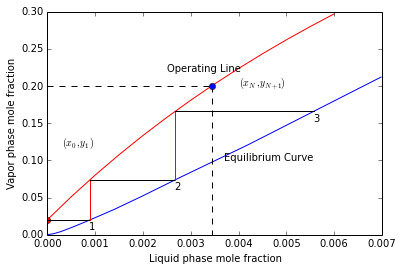## Example 10.6-2 Page Number 621¶

In :
#Absorption of Acetone in a PAcked Tower
from scipy.optimize import root
import numpy as np
import matplotlib.pyplot as plt

#Variable Declaration
P, T= 101325., 293.   #column pressure and temperature
y1, y2 = 0.026, 0.005 #Compositions in gas phase
x2 = 0.0              #Composition of fresh solvent feed
V, L = 13.65, 45.36   #Vapor and liquid rates to the coloumn
kyd, kxd = 3.78e-2, 6.16e-2  #Film coefficient for gas and liquid phase, (kmol/(s.m3.mol frac))
xA, pA, PmmHg = 0.0333, 30., 760.   #liquid equilibrium mole frac at partial presure
A = 0.186              #Tower cross sectional area
#Calculations
def func1(s1,c1,s2,c2,s3,x0,y0):
c3 = c1 - (s3-s1)*x0
x = (c3 - c2)/(s2 - s3)
y = s2*x + c2
return [x,y]

def lm(a,b,c,d):
return ((a-b)-(c-d))/log((a-b)/(c-d))

S = (pA/PmmHg)/xA
k1 = V*y1/(1-y1)-V*y2/(1-y2)
k1 = k1/L
x1 = k1/(1+k1)
yA = pA/PmmHg
me = yA/xA
ce = 0.0
mop = (y2-y1)/(x2-x1)
cop = y2 - mop*x2
xe = np.arange(0.,0.015,0.001)
ye = me*xe
plt.plot(xe,ye,'b-')
plt.plot([x1,x2], [y1,y2], 'ko-', lw=0.5)
m1  = -(kxd/(1-x1))/(kyd/(1-y1))
m2  = -(kxd/(1-x2))/(kyd/(1-y2))
[xi1,yi1]=func1(mop,cop,me,ce,m1,x1,y1)
plt.plot([x1,xi1], [y1,yi1], 'ko-', lw=0.5)
[xi2,yi2]=func1(mop,cop,me,ce,m2,x2,y2)
plt.plot([x2,xi2], [y2,yi2], 'ko-', lw=0.5)

plt.text(.006, .006, 'Equilibrium Curve')
plt.text(.001, .02, 'Operating Line')

plt.annotate('$(x_1,y_1)$', xy=(x1,y1), xytext=(x1,y1+0.002),
arrowprops=dict(facecolor='black', shrink=0.05),
)

plt.annotate('$(x_2,y_2)$', xy=(0,y2), xytext=(0.0005,y2),
arrowprops=dict(facecolor='black', shrink=0.05),
)
plt.xlabel("Liquid phase mole fraction, $x$")
plt.ylabel("Vapor phase mole fraction, $y$")
plt.plot([0,x1,x1], [y1,y1,0], 'ko-', lw=0.5)
plt.plot([0,xi1,xi1], [yi1,yi1,0], 'ko-', lw=0.5)
plt.plot([0,xi2,xi2], [yi2,yi2,0], 'ko-', lw=0.5)
V1 = V/(1-y1)
V2 = V/(1-y2)
V = (V1+V2)*.5/3600.
L = L/3600.
dyyilm = lm(y1,yi1,y2,yi2)
zy = V*(y1-y2)/(kyd*dyyilm*A)
dxxilm = lm(xi1,x1,xi2,x2)
zx = L*(x1-x2)/(kxd*dxxilm*A)
y1s = me*x1
y2s = me*x2
plt.plot([0,x1,x1], [y1s,y1s,0], 'ko-', lw=0.5)
d1yilm1 = lm(1.,yi1,1.,y1)
d1yilm2 = lm(1.,yi2,1.,y2)
d1yilm = (d1yilm1 + d1yilm2)/2.
d1xilm = lm(1.,x1,1.,xi1)
d1ys1lm = lm(1.,y1s,1.,y1)
d1ys2lm = lm(1.,y2s,1.,y2)
d1yslm = (d1ys1lm + d1ys2lm)/2.
Kya = 1./(d1yslm*(1/(kyd/d1yilm) + me/(kxd/d1xilm)))
dyyslm = lm(y1,y1s,y2,y2s)
z = V*(y1-y2)/(A*Kya*dyyslm)
#Results
print "The values of kya, kxa, Kya are:", round(kyd,4),round(kxd,4),round(Kya,4)," kgmol/(s.m2. mol frac) respectively"
print "Tower Height calculated using kya:", round(zy,4),'m'
print "Tower Height calculated using kxa:", round(zx,4),'m'
print "Tower Height calculated using Kya:", round(z,4),'m'

The values of kya, kxa, Kya are: 0.0378 0.0616 0.0223  kgmol/(s.m2. mol frac) respectively
Tower Height calculated using kya: 1.9489 m
Tower Height calculated using kxa: 1.9394 m
Tower Height calculated using Kya: 1.8973 m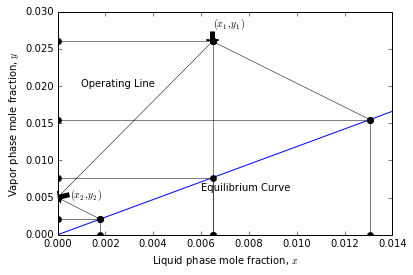## Example 10.6-3 Page Number 625¶

In :
#Use of Transfer Unit for Packed Tower

#Variable Declaration
kyd = 0.0378        #Film coefficient in kmol/s.m3
kxd = 0.0616        #Film coefficient in kmol/s.m3
Kya = 0.022523      #Overall mass transfer coefficient
V = 3.852e-3        #Molar flowrate kmol/s
A = 0.186           #Cross sectional area of the tower m2
y1 = 0.026
y2 = 0.005
#From answers to previous problem
yi1 = 0.0154
yi2 = 0.0020
d1yilm = 0.97925
d1yilm1 = 0.979249088369
d1yilm2 = 0.996443506323
d1xilm = 0.99023
d1yslm = 0.98313
dyyilm = 0.00590
d1yslm = 0.983131759622
d1ys2m = 0.997497911442
dyyslm = 0.0102576937583

#Calculations
#PART A
HG = V/(kyd*A)
NG1 = (d1yilm1/(1-y1) + d1yilm2/(1-y2) )/2.
NG2 = (y1-y2)/dyyilm
NG = NG1*NG2
z = HG*NG
#Results
print "Answers to Part A"
print "Height of gas transfer unit",round(HG,3)
print "Number of gas transfer unit",round(NG,3)
print "Total Height of tower", round(z,4),'m'

#PART B
HoG = V/(Kya*A)
NoG1 = (d1ys1lm/(1-y1) + d1ys2lm/(1-y2))/2.
NoG2 = (y1-y2)/dyyslm
NoG = NoG1*NoG2
z = HoG*NoG

#Results
print "Answers to Part B"
print "Height of Overall gas transfer unit",round(HoG,3)
print "Number of Overall gas transfer unit",round(NoG,3)
print "Total Height of tower", round(z,4),'m'

Answers to Part A
Height of gas transfer unit 0.548
Number of gas transfer unit 3.571
Total Height of tower 1.9567
Answers to Part B
Height of Overall gas transfer unit 0.919
Number of Overall gas transfer unit 2.059
Total Height of tower 1.8936


## Example 10.7-1 Page Number 628¶

In :
#Desing of an Absorption Tower with Concentrated Gas Mixture
from scipy.interpolate import interp1d
import numpy as np
import scipy.integrate as integrate

#Variable Declaration
dp = 0.0254       #Diameter of the rings ,m
x2 = 0.0
P = 101325        #Total pressure ,Pa
y1 = 0.20         #Inlet Mole fraction of SO2
y2 = 0.02         #Outlet Mole fraction of SO2
Vd = 6.53e-4      #Molar flowrate of air ,kmol/s
Ld = 4.20e-2      #Molar flowrate of water ,kmol/s
AT = 0.0929       #Tower cross sectional area,m2
xe = np.array([0.0,0.0000562,0.0001403,0.000280,0.000422,0.000564,0.000842,0.001403,0.001965,0.00279,0.00420,0.00698])
ye = np.array([0.0,0.000658,0.00158,0.00421,0.00763,0.0112,0.01855,0.0342,0.0513,0.0775,0.121,0.212])
yop = np.array([0.02,0.04,0.07,0.13,0.20])
xop = np.zeros(len(yop))
Gy = np.zeros(len(yop))
Gx = np.zeros(len(yop))
V = np.zeros(len(yop))
L = np.zeros(len(yop))
kyda = np.zeros(len(yop))
kxda = np.zeros(len(yop))
xi = np.array([0.00046,0.00103,0.00185,0.00355,0.00565])
yi = np.array([0.0090,0.0235,0.0476,0.1015,0.1685])
d1y = 1.-yop
dyyi = yop-yi
d1yyilm = ((1.-yop)-(1.-yi))/log((1.-yop)/(1.-yi))

#Calcualations
def funkxda(gx):
return 0.152*gx**0.82

def funkyda(gx,gy):
return 0.0594*gx**0.25*gy**0.7

k1 = (Ld*x2/(1.-x2)+Vd*(y1/(1.-y1)-y2/(1.-y2)))/Ld
x1 = k1/(1.+k1)

for j in range(len(yop)):
k1 = (Ld*x1/(1.-x1)+Vd*(-y1/(1.-y1)+yop[j]/(1.-yop[j])))/Ld
xop[j] = k1/(1+k1)
Gy[j] = (Vd*29.+Vd*yop[j]*64.1/(1.-yop[j]))/AT
Gx[j] = (Ld*18.+Ld*xop[j]*64.1/(1.-xop[j]))/AT
V[j] = Vd/(1.-yop[j])
L[j] = Ld/(1.-xop[j])
kxda[j] = funkxda(Gx[j])
kyda[j] = funkyda(Gx[j],Gy[j])

fyop = V/((kyda*AT/d1yyilm)*d1y*dyyi)
Height = integrate.simps(fyop,yop)

#Results
print "Height of the tower:",round(Height,3), "m"

Height of the tower: 1.574 m


## Example 10.8-1 Page Number 633¶

In :
#Prediction of Film Coefficients for Ammonia Absorption

#Variable Declaration
T = 303            #Temperature of the tower in K
dp = .0254         #Diameter of the ring m
P = 101325         #Total pressure in Pa
Gx = 2.543         #Flowrate in kg/s.m2
Gy = 0.339         #Flowrate in kg/s.m2
m = 1.2
mua = 1.86e-5      #Viscosity of air in Pa.s
rhoa = 1.168       #Density of air in kg/m3
DAB = 2.379e-5     #Diffusivity of NH3 in water , m2/s
alpha = 0.557      #Constant in correlation for gas phase mass transfer coefficient
beta = 0.32        #Constant in correlation for gas phase mass transfer coefficient
gama = -0.51       #Constant in correlation for gas phase mass transfer coefficient
muw15 = 1.1404e-3  #Viscosity of water at 15°C
muw30 = 0.8007e-3  #Viscosity of water at 30°C
DABw15 = 1.77e-9   #Diffusivity of NH3 in water at 15°C
rhow = 996.        #Desity of water in kg/m3
theta = 2.35e-3    #Constant in correlation for liquid phase mass transfer coefficient
eta = 0.22         #Constant in correlation for liquid phase mass transfer coefficient
#Calcualations
V = Gy/(29.0)
L = Gx/18.0
Sca = mua/(rhoa*DAB)
HG = alpha*Gy**beta*Gx**gama*Sca**0.5
DABw = muw15*303/(muw30*288)*DABw15
Scw = muw30/(rhow*DABw)
HL = theta*(Gx/muw30)**eta*Scw**0.5
kyda = V/HG
kxda = L/HL
Kyda = 1./(1./kyda + m/kxda)
ResGf = (1./kyda)/(1./Kyda)*100

#Results
print "Predicted film coefficients are as follows "
print "Gas phase film cofficient ",round(kyda,4),"kmol/(s.m3.mol frac)"
print "Liquid phase film cofficient ",round(kxda,3),"kmol/(s.m3.mol frac)"
print "Overall Gas phase film cofficient ",round(Kyda,4),"kmol/(s.m3.mol frac)"
print "Percentage resistance in gas film is ",round(ResGf,1), "%"

Predicted film coefficients are as follows
Gas phase film cofficient  0.0584 kmol/(s.m3.mol frac)
Liquid phase film cofficient  0.586 kmol/(s.m3.mol frac)
Overall Gas phase film cofficient  0.0521 kmol/(s.m3.mol frac)
Percentage resistance in gas film is  89.3 %